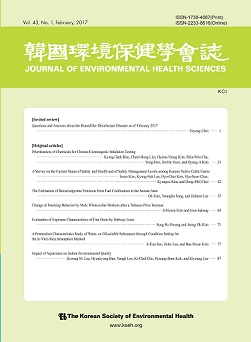상세검색
최근 검색어 전체 삭제
다국어입력
즐겨찾기0KCI우수등재 학술저널

# 일부 농약의 가수분해속도상수의 측정Determination of Hydrolysis Rate Constants in some Pesticides

• 등재여부 : KCI우수등재
• 1999.06
• 97 - 106 (10 pages)
• 3

The present study was performed to determine the hydrolysis rate constants and degradation products of BPMC, chlorothalonil, dichlorvos and methidathion by the OECD method. The hydrolysis rate was fastest for dichlorvos, followed by methidathion, BPMC and chlorothalonil. Hydrolysis of BPMC, chlorothalonil, dichlorvos and methidathion were instable in alkaline solution(pH 9) at 25 and 40°C. Hydrolysis rate constants of BPMC in pH 4, pH 7, and pH 9 buffer solutions at 25 and 40°C were 0.0025, 0.0028, 0.0799 and 0 .0037, 0.0055, 0.3001, respectively. Hydrolysis rate of BPMC in pH 9 at 40°C was 3 times faster than that at 25°C. Hydrolysis rate constants of chlorothalonil in pH 4, pH 7, and pH 9 buffer solutions at 25 and 40°C were 0.0013, 0.0017, 0.0199 and 0.0014, 0.0028, 0.2459, respectively. Hydrolysis rate of chlorothalonil in pH 9 at 40°C was 15 times faster than that at 25°C. Hydrolysis rate constants of dichlorvos in pH 4, pH 7, and pH 9 buffer solutions at 25 and 40°C were 0 .1123, 0.4233, 0.7123 and 0.4091, 1.6132, 3.0829, respectively. Hydrolysis rate of dichlorvos was fast under all conditions, half-life was within 6 days. Hydrolysis rate constants of methidathion in pH 4, pH 7, and pH 9 buffer solutions at 25 and 40°C were 0.0310, 0 .0355, 0.0901 and 0.4581, 0.4898, 1.0726, respectively. Hydrolysis rate of methidathion in pH 4, pH 7 , and pH 9 at 40°C was 13 times faster than that at 25°C . It is suggested that hydrolysis rate of methidathion mainly depends on temperature than pH. In order to identify hydrolysis products, the extracts of degradation products were analyzed by GC/MS. Degradation products of BPMC and chlorothalonil were not identified in hydrolysis tests. The mass spectrum of hydrolysis products of dichlorvos was at m/z 131, that of the hydrolysis of methidathion was at m/z 192.

I. 서론

II. 실험재료 및 방법

III. 결과 및 고찰

IV. 결론